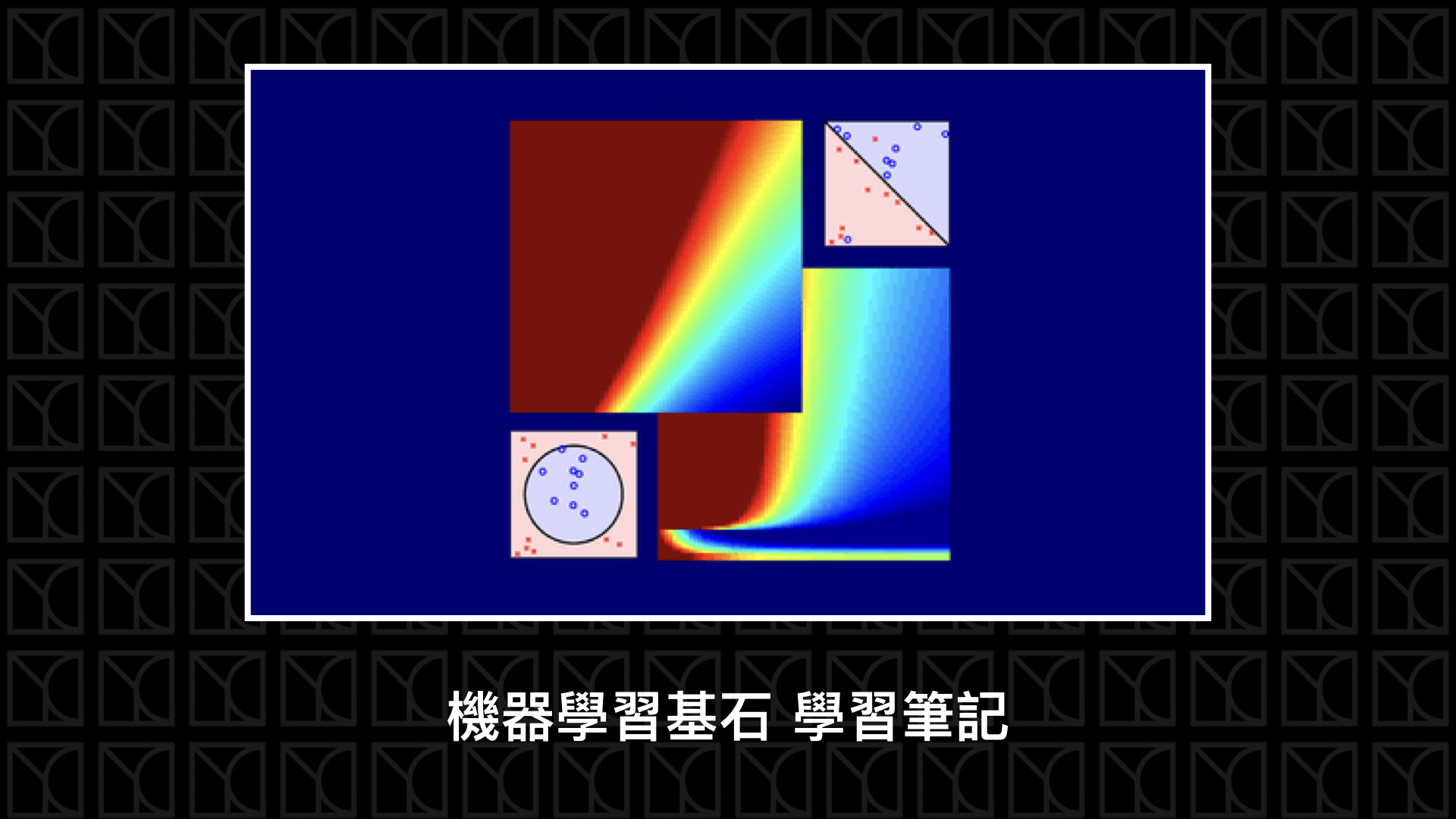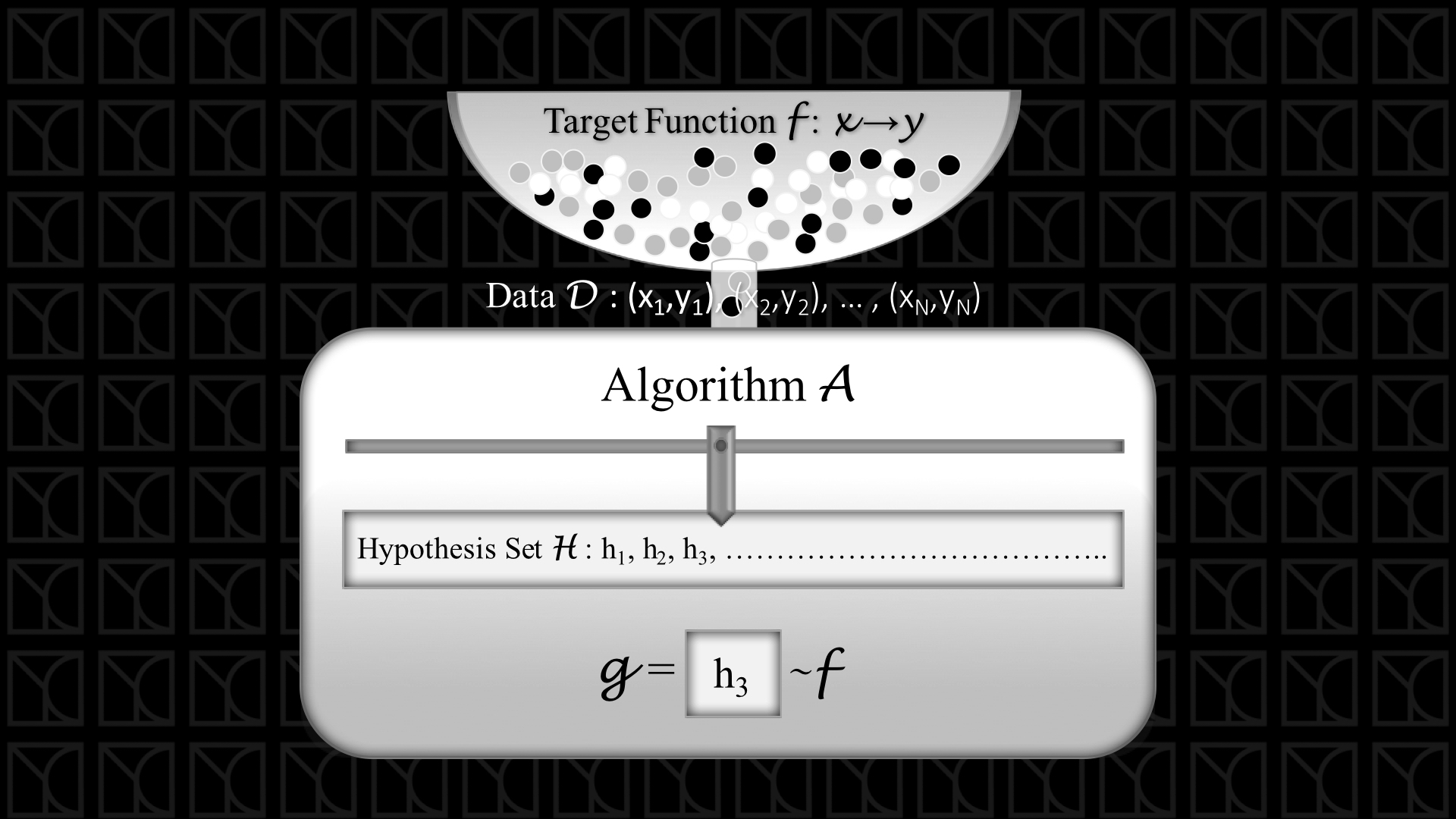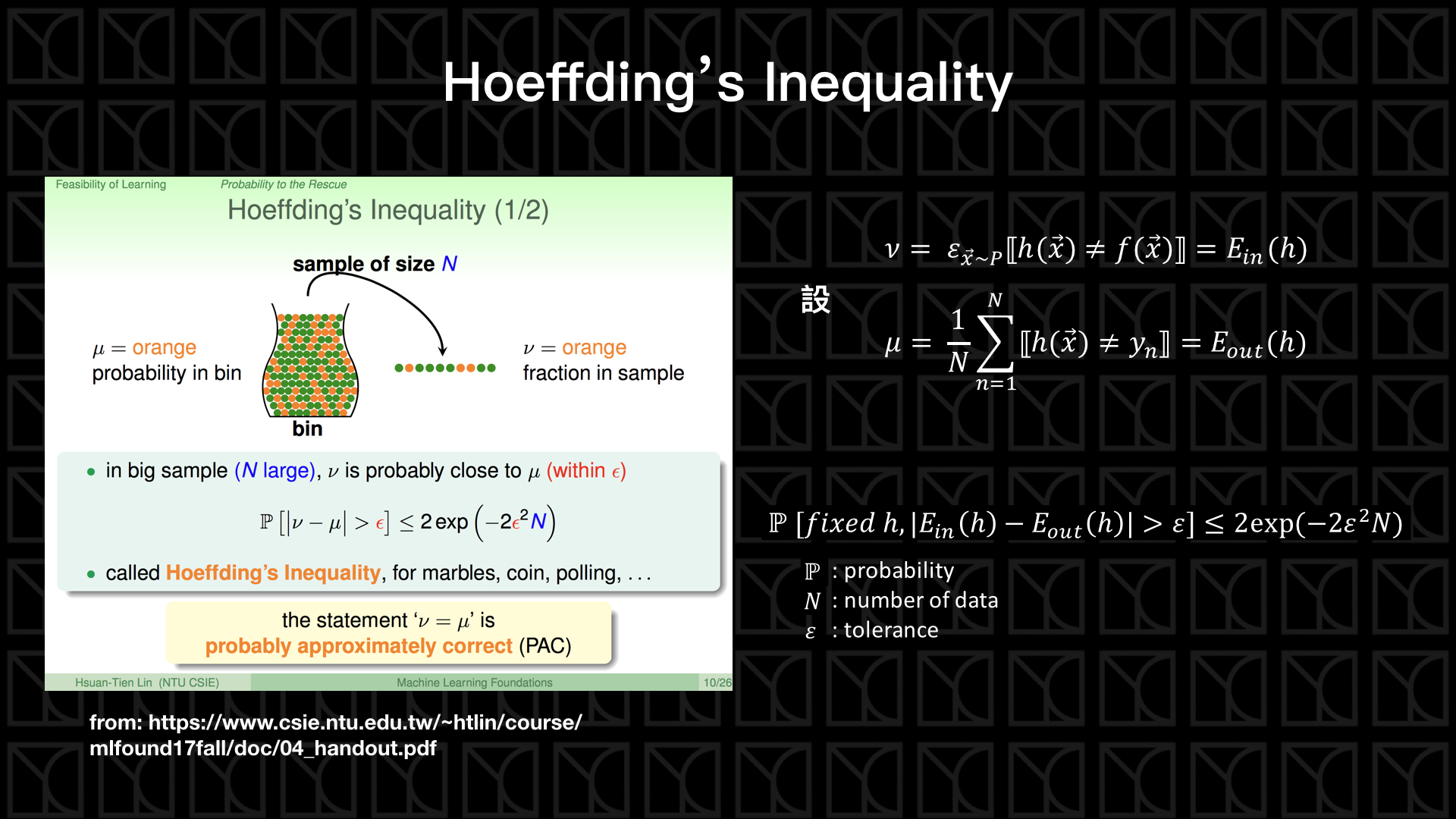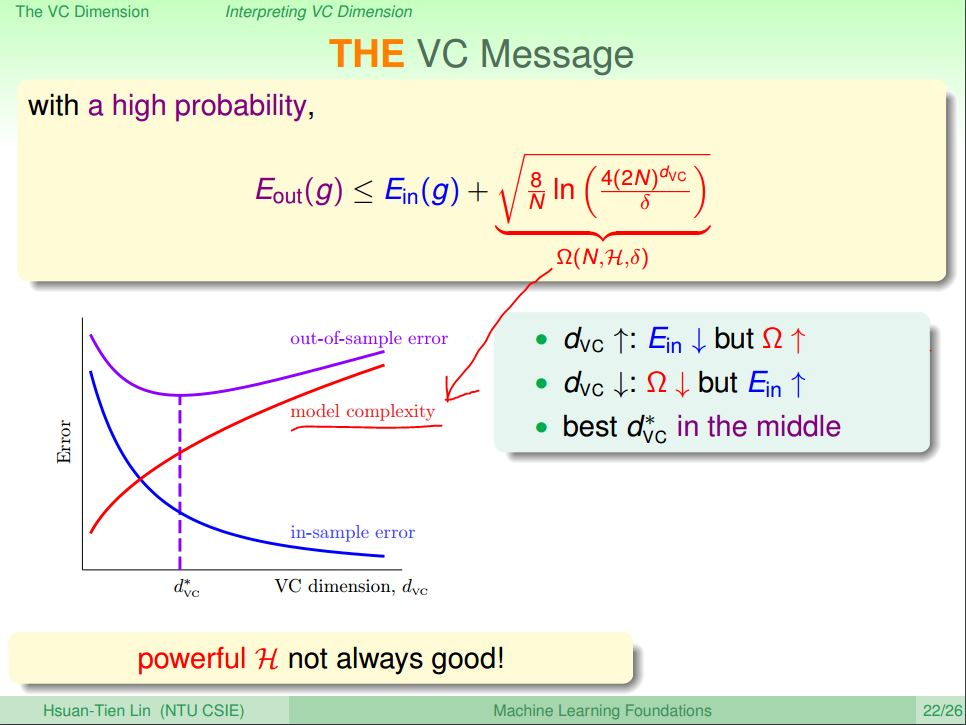# 機器學習基石 學習筆記 (2)：為什麼機器可以學習?

### 機器可以學習嗎?### $$E_{in}$$和$$E_{out}$$的差異$$\mathbb{P}[|ν-μ|>ε] \leq 2 exp(-2ε^2N)$$

$$ε$$越大，出現的機率就越低。

$$μ = (1/N) \sum_{n=1}^N ⟦h(x)\neq y_n⟧ = E_{in}(h)$$

$$ν = ε_{x \sim P} ⟦h(x)\neq f(x)⟧ = E_{out}(h)$$

$$\mathbb{P}[|E_{in}(h)-E_{out}(h)|>ε] \leq 2 exp(-2ε^2N)$$$$\mathbb{P}[\exists h\in \mathbb{H}\ s.t.\ |E_{in}(h)-E_{out}(h)|>ε] \leq 2M exp(-2ε^2N)$$

### VC Generalization Bound$$\mathbb{P}[\exists h\in \mathbb{H}\ s.t.\ |E_{in}(h)-E_{out}(h)|>ε] \leq 4m_{\mathbb{H}}(2N) exp(-ε^2N/8)$$

，原本的$$M$$消失了，取而代之的是Growth Function $$m_{\mathbb{H}}(2N)$$，Growth Function與Data數量$$N$$有關，這就是我們剛剛解說的，決定Hypothesis Set的種類的其實是 Data的數量$$N$$

$$m_{\mathbb{H}}(n) = n^{d_{VC}},\ d_{VC} = BreakPoint-1$$

### 機器要能學習的三要素

1. Good Hypothesis Set: Hypothesis Set 必須有Break Point的存在，也意味著VC Dimension是有限的，而且越小越好，在意義上代表可以調控的變數不要太多。
2. Good Data: 數據量越大越好，可以壓低VC Generalization Bound
3. Good Learning Algorithm: 以上兩點可以確定的是$$E_{in} \approx E_{out}$$，接下來好的Learning Algorithm要有能力找到$$E_{in}$$ 最小的參數。很直觀的，當我們可以調控的變數越多，我們的選擇就越多，也就是我們可以找到更小$$E_{in}$$ 的機會變多了，所以可以調控的變數不可以太少。### 機器學習架構一般化1. 每筆Data出現的機會不一定，同樣的採樣結果也是會受機率的影響，所以上圖中標示為$$\mathbb{P} (x)$$，這個修改並不會影響機器學習的流程和結果。
2. Data可能會受到Noise的影響，所以給定$$X_n$$並不一定會百分之一百得到$$y_n$$，他存在著可能會出錯，上圖標示為$$\mathbb{P}(y|x)$$，我們可以增大我們採樣的數量$$N$$來減少Noise的影響。
3. 我們是採用$$E_{in}$$來當作選擇Model參數的指標，因此我們需要訂出Error的評估方式，常見的有Squared Error $$E_{squared} = (y_n - y_{prediction})^2$$

1. 準備好足夠的數據
2. 把Model建立好，$$d_{VC}$$必須要是有限的，而且大小要適中
3. 定義好評估$$E_{in}$$的Error Measurement
4. 使用演算法找出最佳參數把$$E_{in}$$降低
5. 最後評估一下是否有Overfitting的狀況，確保$$E_{in} \approx E_{out}$$（未來會講怎麼做）